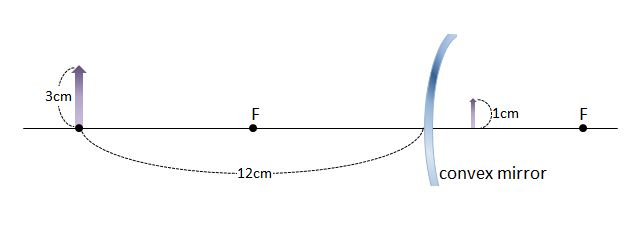# Convex mirrorAs shown above, when an object with a height of $3\text{ cm}$ is placed on the optical axis of a convex mirror at a distance of $12\text{ cm}$ from the mirror, the height of its image is $1\text{ cm}.$ Then what is the focal length of the convex mirror?

×